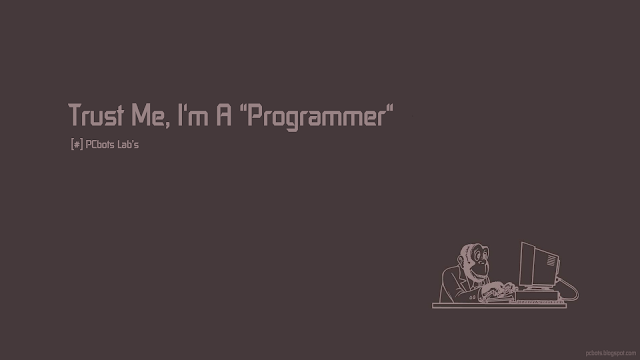Hey friends! My name is yuvraj basnet. I'm going to introduce myself.I have born and grown up in india(chandigarh) and I'm studying second year of B.C.A computer science field. My father serving in indian air force. My mother has been taking care of house hold. I am a friendly person, good thinker, I can work for longer hours without any break, only if I'm interested in that...My life style is very simple.

# DECISION MAKINGDECISION MAKING

C programming is a set of statements or set of instructions, which are normally executed from the order in which they appear or written. This happens when no certain option or certain condition is used in programming.

There may be a situations where we need to change the order of execution of statements and repeat execution of certain statements depending on conditions. That's why we use decision making and control statements.

C language support the following decision statements:

1) if statement
2) switch statement
3) Conditional Operator statement
4) goto statement

Decision making with if statements-
The statement may be implemented in different forms depending on the complexity of conditions to be tested. The different forms are
A) Simple if statement
B) if else statement
C) Nested if else statement
A) Simple if statement-
An if statement consists of a boolean expression followed by one or more statements.

Example
``````
void main( )
{
int A, B;
A = 15;
B = 13;
if (A > B )
{
printf(" A is greater than B");
}
}``````

OUTPUT-
A is greater than B.

B) if else statement-
An if statement can be followed by an optional else statement, which executes when the Boolean expression is false.

Example
``````void main( )
{
int A, B;
A = 15;
B= 18;
if (A > B )
{
printf("A is greater than B");
}
else
{
printf("B is greater than A");
}
}``````

OUTPUT-
B is greater than A.

C) Nested if else statement-
It is possible to include if else statement(s) inside the body of another
if else statement.

Example
``````#include <stdio.h>

void main( )
{
int x, y, z;
printf("Enter 3 numbers...");
scanf("%d%d%d",&x, &y, &z);
if(x > y)
{
if(x > z)
{
printf("x is the greatest");
}
else
{
printf("z is the greatest");
}
}
else
{
if(y > z)
{
printf("y is the greatest");
}
else
{
printf("z is the greatest");
}
}
}``````

It's used in a program when if statement having multiple decisions.

Example
``````oid main( )
{
int x;
printf("Enter a number...");
scanf("%d", &x);
if(x%5 == 0 && x%8 == 0)
{
printf("Divisible by both 5 and 8");
}
else if(x%8 == 0)
{
printf("Divisible by 8");
}
else if(x%5 == 0)
{
printf("Divisible by 5");
}
else
{
printf("Divisible by none");
}
}``````

2) SWITCH STATEMENT-
Switch statement is a control statement that allows us to choose only one choice among the many given choices.

It executes that block of code which matches the case value. If there is no match, then default block is executed(if present).

Example
``````void main( )
{
int x, y, z, choice;
while(choice != 3)
{
printf("\n 1. Press 1 for addition");
printf("\n 2. Press 2 for subtraction");
scanf("%d", &choice);

switch(choice)
{
case 1:
printf("Enter 2 numbers");
scanf("%d%d", &x, &y);
z = x + y;
printf("%d", z);
break;
case 2:
printf("Enter 2 numbers");
scanf("%d%d", &x, &y);
z = x - y;
printf("%d", z);
break;
default:
printf("you have passed a wrong key");
}
}
} ``````

3) CONDITIONAL OPERATOR STATEMENT-
• Conditional operators return one value if condition is true and returns another value is condition is false.
• This operator is also called as ternary operator.
Syntax :  (Condition? true_value: false_value);

Example
int main()
{
int x=1, y ;
y = ( x ==1 ? 2 : 0 ) ;
printf("x value is %d\n", x);
printf("y value is %d", y);
}

OUTPUT-
X value is 1
Y value is 2

4)GOTO STATEMENT-
The goto statement is a jump statement which is sometimes also referred to as unconditional jump statement. The goto statement can be used to jump from anywhere to anywhere within a function
```